+关注继续查看

# 第14章 循环神经网络

## 循环神经元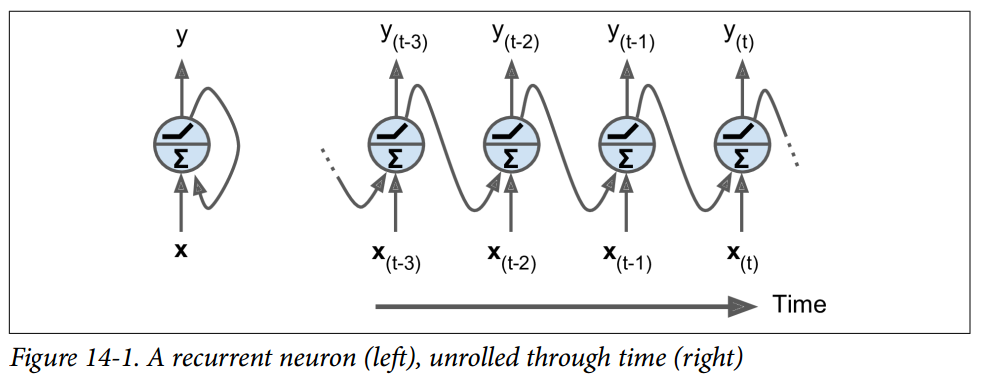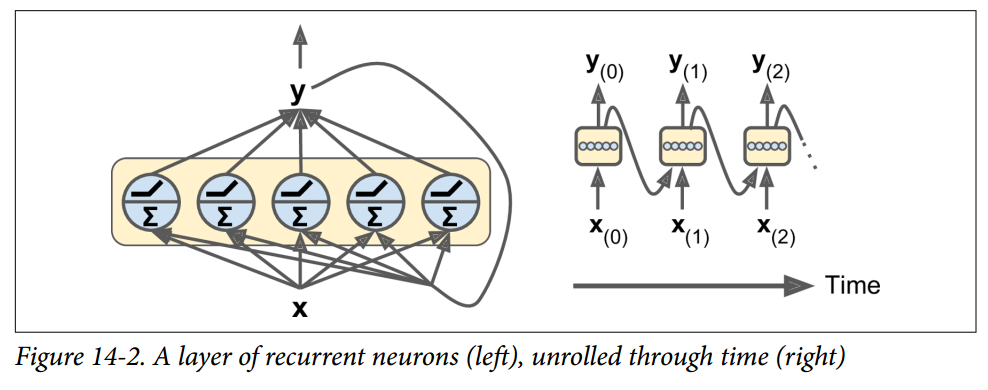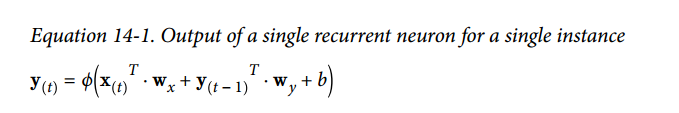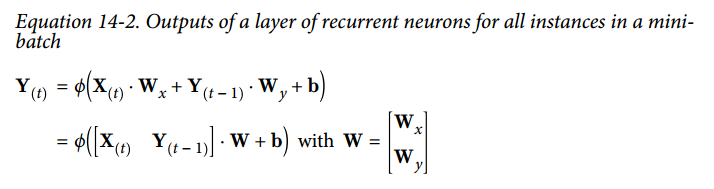• Y(t)Y(t)是m \times n_{neurons}tm矩阵，包含在最小批次中每个实例在时间步‘t‘处的层输出（‘m‘是小批次中的实例数，n_{neurons}\$ 是神经元数）。
• X(t)X(t)m×ninputsm×ninputs 矩阵，包含所有实例的输入的 （ninputsninputs 是输入特征的数量）。
• WxWxninputs×nneuronsninputs×nneurons 矩阵，包含当前时间步的输入的连接权重的。
• WyWynneurons×nneuronsnneurons×nneurons 矩阵，包含上一个时间步的输出的连接权重。
• 权重矩阵 WxWxWyWy 通常连接成单个权重矩阵W，形状为 (ninputs+nneurons)×nneurons(ninputs+nneurons)×nneurons（见公式 14-2 的第二行）
• b是大小为 nneuronsnneurons 的向量，包含每个神经元的偏置项。

## 记忆单元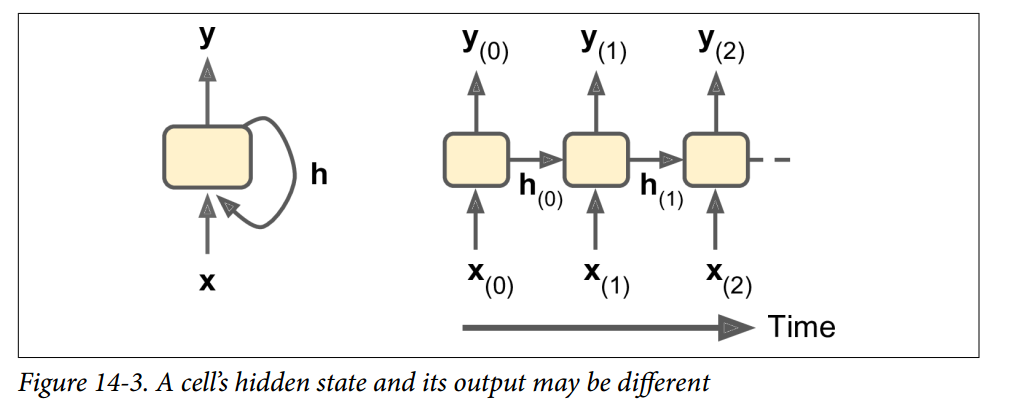## 输入和输出序列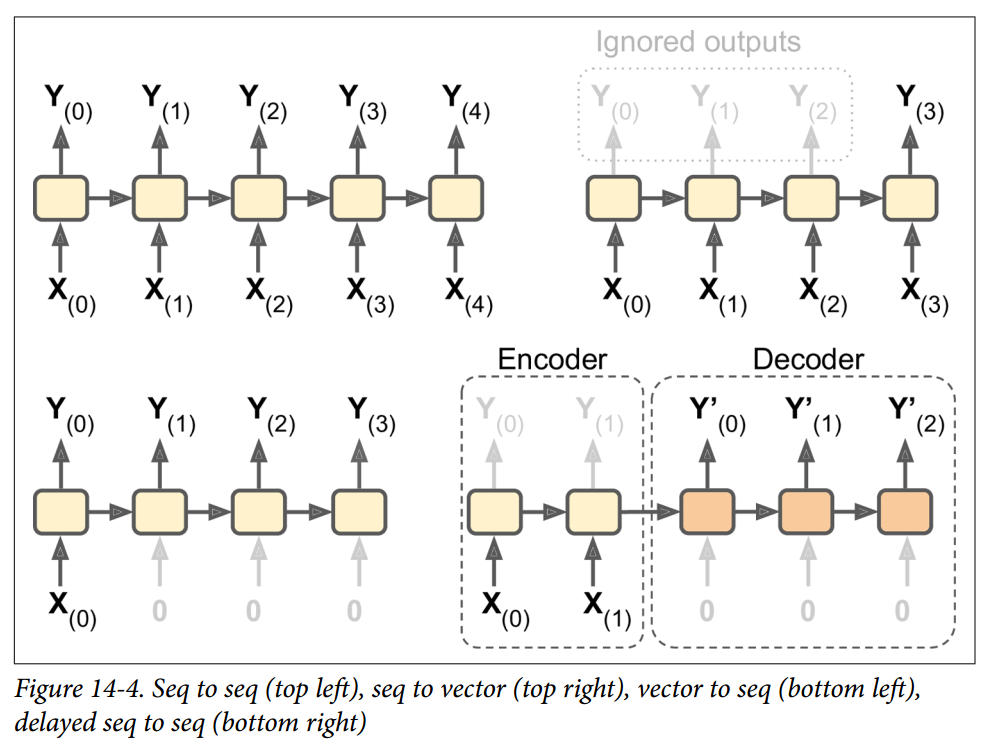RNN 可以同时进行一系列输入并产生一系列输出（见图 14-4，左上角的网络）。 例如，这种类型的网络对于预测时间序列（如股票价格）非常有用：你在过去的N天内给出价格，并且它必须输出向未来一天移动的价格（即从N - 1天前到明天）。

## TensorFlow 中的基本 RNN

n_inputs = 3
n_neurons = 5
X0 = tf.placeholder(tf.float32, [None, n_inputs])
X1 = tf.placeholder(tf.float32, [None, n_inputs])
Wx = tf.Variable(tf.random_normal(shape=[n_inputs, n_neurons], dtype=tf.float32))
Wy = tf.Variable(tf.random_normal(shape=[n_neurons, n_neurons], dtype=tf.float32))
b = tf.Variable(tf.zeros([1, n_neurons], dtype=tf.float32))
Y0 = tf.tanh(tf.matmul(X0, Wx) + b)
Y1 = tf.tanh(tf.matmul(Y0, Wy) + tf.matmul(X1, Wx) + b)
init = tf.global_variables_initializer()

    # Mini-batch: instance 0,instance 1,instance 2,instance 3
X0_batch = np.array([[0, 1, 2], [3, 4, 5], [6, 7, 8], [9, 0, 1]])  # t = 0
X1_batch = np.array([[9, 8, 7], [0, 0, 0], [6, 5, 4], [3, 2, 1]])  # t = 1
with tf.Session() as sess:
init.run()
Y0_val, Y1_val = sess.run([Y0, Y1], feed_dict={X0: X0_batch, X1: X1_batch})

>>> print(Y0_val) # output at t = 0
[[-0.2964572 0.82874775 -0.34216955 -0.75720584 0.19011548] # instance 0
[-0.12842922 0.99981797 0.84704727 -0.99570125 0.38665548] # instance 1
[ 0.04731077 0.99999976 0.99330056 -0.999933 0.55339795] # instance 2
[ 0.70323634 0.99309105 0.99909431 -0.85363263 0.7472108 ]] # instance 3
>>> print(Y1_val) # output at t = 1
[[ 0.51955646 1\. 0.99999022 -0.99984968 -0.24616946] # instance 0
[-0.70553327 -0.11918639 0.48885304 0.08917919 -0.26579669] # instance 1
[-0.32477224 0.99996376 0.99933046 -0.99711186 0.10981458] # instance 2
[-0.43738723 0.91517633 0.97817528 -0.91763324 0.11047263]] # instance 3

import numpy as np
import tensorflow as tf

if __name__ == '__main__':
n_inputs = 3
n_neurons = 5
X0 = tf.placeholder(tf.float32, [None, n_inputs])
X1 = tf.placeholder(tf.float32, [None, n_inputs])
Wx = tf.Variable(tf.random_normal(shape=[n_inputs, n_neurons], dtype=tf.float32))
Wy = tf.Variable(tf.random_normal(shape=[n_neurons, n_neurons], dtype=tf.float32))
b = tf.Variable(tf.zeros([1, n_neurons], dtype=tf.float32))
Y0 = tf.tanh(tf.matmul(X0, Wx) + b)
Y1 = tf.tanh(tf.matmul(Y0, Wy) + tf.matmul(X1, Wx) + b)
init = tf.global_variables_initializer()

# Mini-batch: instance 0,instance 1,instance 2,instance 3
X0_batch = np.array([[0, 1, 2], [3, 4, 5], [6, 7, 8], [9, 0, 1]])  # t = 0
X1_batch = np.array([[9, 8, 7], [0, 0, 0], [6, 5, 4], [3, 2, 1]])  # t = 1
with tf.Session() as sess:
init.run()
Y0_val, Y1_val = sess.run([Y0, Y1], feed_dict={X0: X0_batch, X1: X1_batch})

print(Y0_val,'\n')
print(Y1_val)

## 时间上的静态展开

static_rnn()函数通过链接单元来创建一个展开的 RNN 网络。 下面的代码创建了与上一个完全相同的模型：

X0 = tf.placeholder(tf.float32, [None, n_inputs])
X1 = tf.placeholder(tf.float32, [None, n_inputs])

basic_cell = tf.contrib.rnn.BasicRNNCell(num_units=n_neurons)
output_seqs, states = tf.contrib.rnn.static_rnn(basic_cell, [X0, X1],
dtype=tf.float32)
Y0, Y1 = output_seqs

X = tf.placeholder(tf.float32, [None, n_steps, n_inputs])
X_seqs = tf.unstack(tf.transpose(X, perm=[1, 0, 2]))

basic_cell = tf.contrib.rnn.BasicRNNCell(num_units=n_neurons)
output_seqs, states = tf.contrib.rnn.static_rnn(basic_cell, X_seqs,
dtype=tf.float32)
outputs = tf.transpose(tf.stack(output_seqs), perm=[1, 0, 2])

X_batch = np.array([
# t = 0      t = 1
[[0, 1, 2], [9, 8, 7]], # instance 1
[[3, 4, 5], [0, 0, 0]], # instance 2
[[6, 7, 8], [6, 5, 4]], # instance 3
[[9, 0, 1], [3, 2, 1]], # instance 4
])

with tf.Session() as sess:
init.run()
outputs_val = outputs.eval(feed_dict={X: X_batch})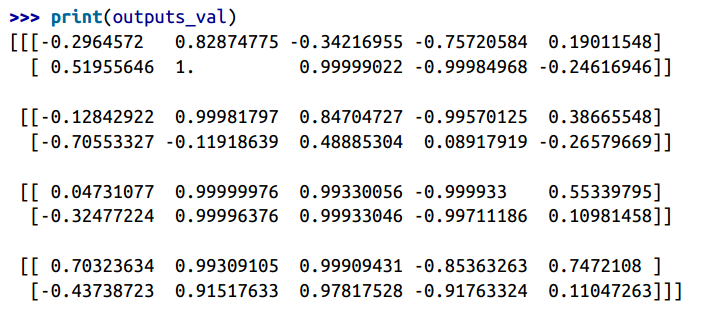## 时间上的动态展开

dynamic_rnn()函数使用while_loop()操作，在单元上运行适当的次数，如果要在反向传播期间将 GPU内 存交换到 CPU 内存，可以设置swap_memory = True，以避免内存不足错误。 方便的是，它还可以在每个时间步（形状为[None, n_steps, n_inputs]）接受所有输入的单个张量，并且在每个时间步（形状[None, n_steps, n_neurons]）上输出所有输出的单个张量。 没有必要堆叠，拆散或转置。 以下代码使用dynamic_rnn()函数创建与之前相同的 RNN。 这太好了！

import numpy as np
import tensorflow as tf
import pandas as pd

if __name__ == '__main__':
n_steps = 2
n_inputs = 3
n_neurons = 5

X = tf.placeholder(tf.float32, [None, n_steps, n_inputs])

basic_cell = tf.contrib.rnn.BasicRNNCell(num_units=n_neurons)
outputs, states = tf.nn.dynamic_rnn(basic_cell, X, dtype=tf.float32)

init = tf.global_variables_initializer()

X_batch = np.array([
[[0, 1, 2], [9, 8, 7]],  # instance 1
[[3, 4, 5], [0, 0, 0]],  # instance 2
[[6, 7, 8], [6, 5, 4]],  # instance 3
[[9, 0, 1], [3, 2, 1]],  # instance 4
])

with tf.Session() as sess:
init.run()
outputs_val = outputs.eval(feed_dict={X: X_batch})

print(outputs_val)

## 处理变长输入序列

n_steps = 2
n_inputs = 3
n_neurons = 5

reset_graph()

X = tf.placeholder(tf.float32, [None, n_steps, n_inputs])
basic_cell = tf.contrib.rnn.BasicRNNCell(num_units=n_neurons)
seq_length = tf.placeholder(tf.int32, [None])
outputs, states = tf.nn.dynamic_rnn(basic_cell, X, dtype=tf.float32,
sequence_length=seq_length)

X_batch = np.array([
# step 0     step 1
[[0, 1, 2], [9, 8, 7]], # instance 1
[[3, 4, 5], [0, 0, 0]], # instance 2 (padded with zero vectors)
[[6, 7, 8], [6, 5, 4]], # instance 3
[[9, 0, 1], [3, 2, 1]], # instance 4
])
seq_length_batch = np.array([2, 1, 2, 2])

with tf.Session() as sess:
init.run()
outputs_val, states_val = sess.run(
[outputs, states], feed_dict={X: X_batch, seq_length: seq_length_batch})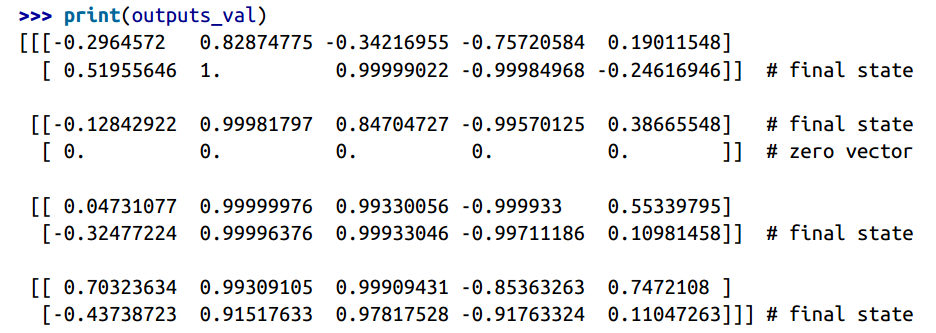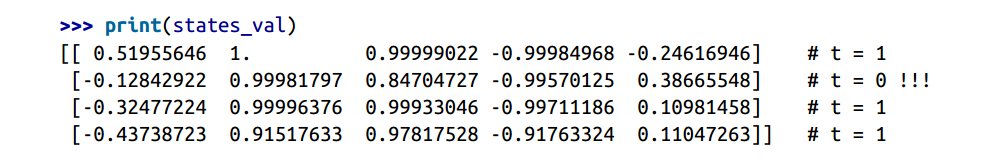## 训练 RNN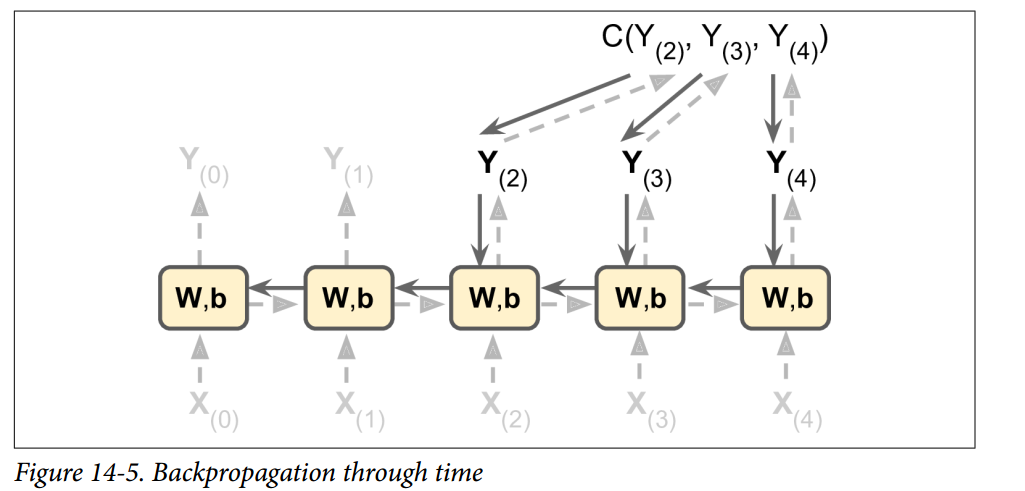## 训练序列分类器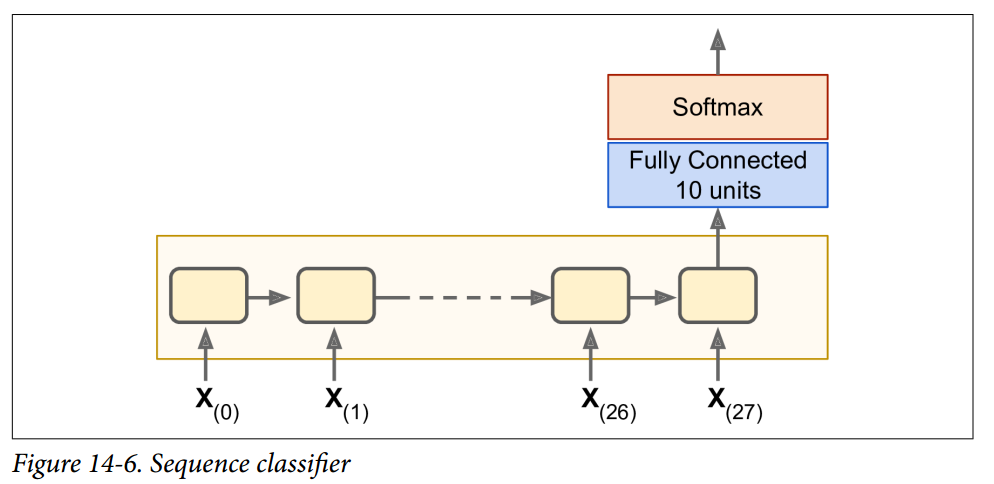n_steps = 28
n_inputs = 28
n_neurons = 150
n_outputs = 10

learning_rate = 0.001

X = tf.placeholder(tf.float32, [None, n_steps, n_inputs])
y = tf.placeholder(tf.int32, [None])

basic_cell = tf.contrib.rnn.BasicRNNCell(num_units=n_neurons)
outputs, states = tf.nn.dynamic_rnn(basic_cell, X, dtype=tf.float32)

logits = tf.layers.dense(states, n_outputs)
xentropy = tf.nn.sparse_softmax_cross_entropy_with_logits(labels=y,
logits=logits)
loss = tf.reduce_mean(xentropy)
init = tf.global_variables_initializer()